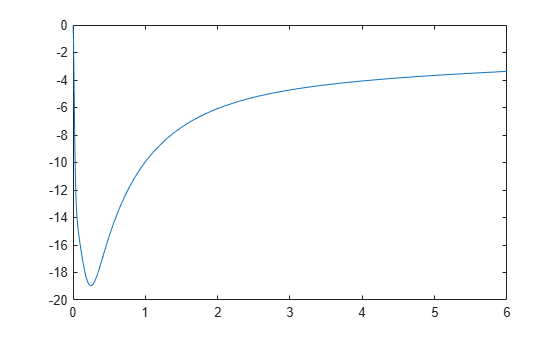# freqresp

Frequency response of a rational function and rationalfit function object

## Syntax

``[response, outputfreq] = freqresp(rationalobject,inputfreq)``
``[response, outputfreq] = freqresp(fit,inputfreq)``

## Description

````[response, outputfreq] = freqresp(rationalobject,inputfreq)` calculates the frequency response, `response` of a rational function object, `rationalobject` at the specified input frequencies, `inputfreq`.```

example

````[response, outputfreq] = freqresp(fit,inputfreq)` calculates the frequency response of the `fit`.```

## Examples

collapse all

Compute the frequency response of data stored in the file,|passive.s2p| by reading it into an `rfdata` object, fitting a rational function object to the data, and using the `freqresp` method to compute the frequency response of the object

`orig_data=read(rfdata.data,'passive.s2p')`
```orig_data = rfdata.data with properties: Freq: [202x1 double] S_Parameters: [2x2x202 double] GroupDelay: [202x1 double] NF: [202x1 double] OIP3: [202x1 double] Z0: 50.0000 + 0.0000i ZS: 50.0000 + 0.0000i ZL: 50.0000 + 0.0000i IntpType: 'Linear' Name: 'Data object' ```
```freq=orig_data.Freq; data=orig_data.S_Parameters(2,1,:); fit_data=rationalfit(freq,data)```
```fit_data = rfmodel.rational with properties: A: [6x1 double] C: [6x1 double] D: 0 Delay: 0 Name: 'Rational Function' ```
```[resp,freq]=freqresp(fit_data,freq); plot(freq/1e9,20*log10(abs(resp)));``````figure plot(freq/1e9,unwrap(angle(resp)));```## Input Arguments

collapse all

Rational function object, specified as an object handle created using `rationalfit`.

Example: ` [resp,freq]=freqresp(fit_data,freq);` where `fit_data` is the rational object handle created using `rationalfit`.

Data Types: `char` | `string`

Input frequencies, specified as a vector of positive frequencies in Hz.

Data Types: `double`

N-by-N array, specified `rfmodel.rational` objects returned by `rationalfit`.

## Output Arguments

collapse all

Frequency response values, returned as a vector.

Frequency values same as input frequencies, returned as a vector.